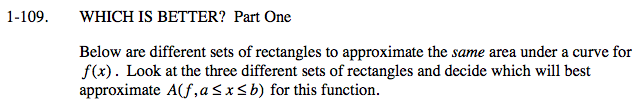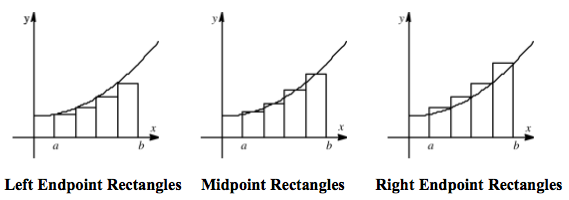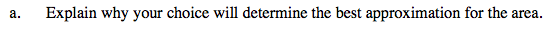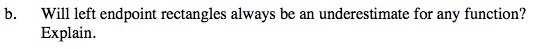### Home > CALC > Chapter Ch1 > Lesson 1.3.1 > Problem1-109

1-109.Which graph is the closest to the exact area under the curve?

Midpoint RectanglesNotice that the graphs in the diagrams above show an increasing function. That is, as x increases, the y-values increase. What if we graphed a decreasing function instead? Would left-endpoint rectangles be underestimates then?